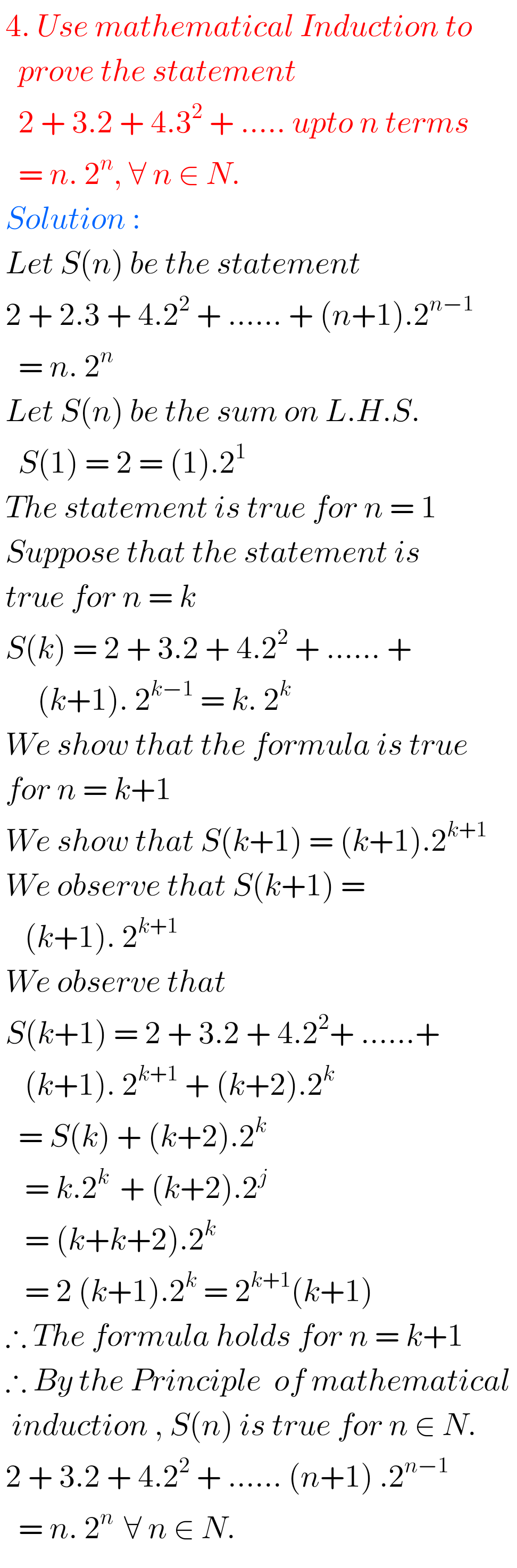## MATHEMATICAL INDUCTION, Intermediate 1st year problems with solutions,solutions for Mathematical Induction Inter first year 1A

MATHEMATICAL INDUCTION, INTERMEDIATE FIRST YEAR PROBLEMS WITH SOLUTIONS Mathematics intermediate first year 1A and 1B solutions for some problems. These solutions are very simple to understand.  Junior inter 1A : Functions, mathematical induction, functions, addition of vectors, trigonometric ratios upto transformations, trigonometric equations, hyperbolic functions, inverse trigonometric functions and properties of triangles. Junior inter 1B : …